# 第四次博客作业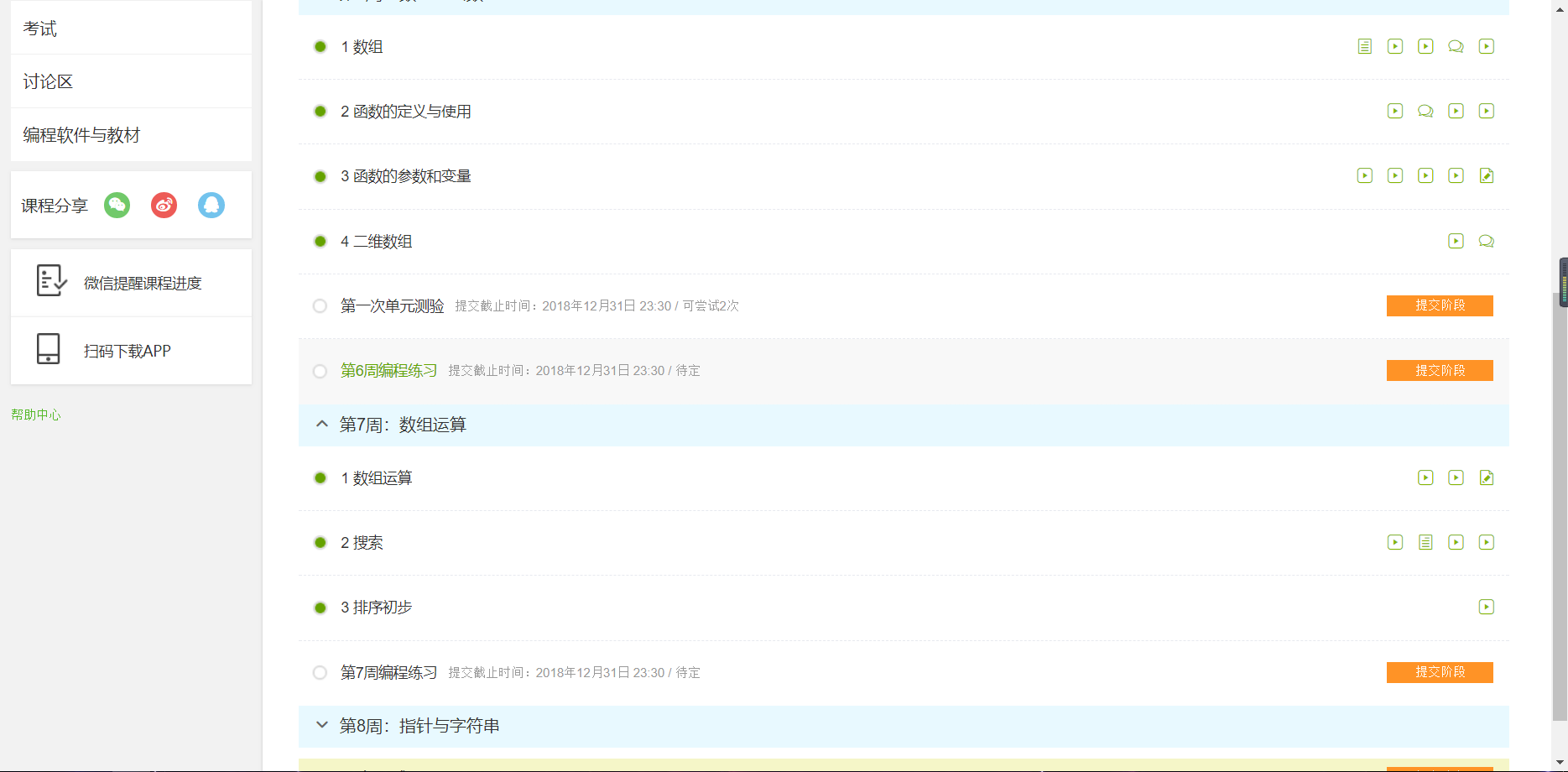#include<stdio.h>
int main()
{
int a,i,count;
double sum=0,average;
scanf("%d",&count);
if(count>=1&&count<=10){
for(i=0;i<count;i++){
scanf("%d",&a[i]);
sum=sum+a[i];
}
average=sum/count;
printf("%.2f\n",average);
if(count==1)printf("\n");
for(i=0;i<count;i++){
if(a[i]>average)printf("%d ",a[i]);
}
}
else
printf("Invalid.");
return 0;
}

解题思路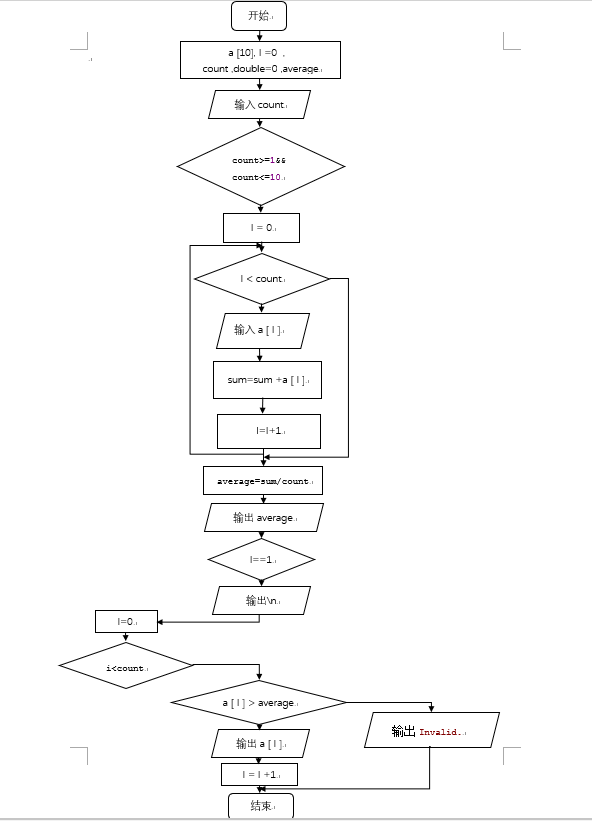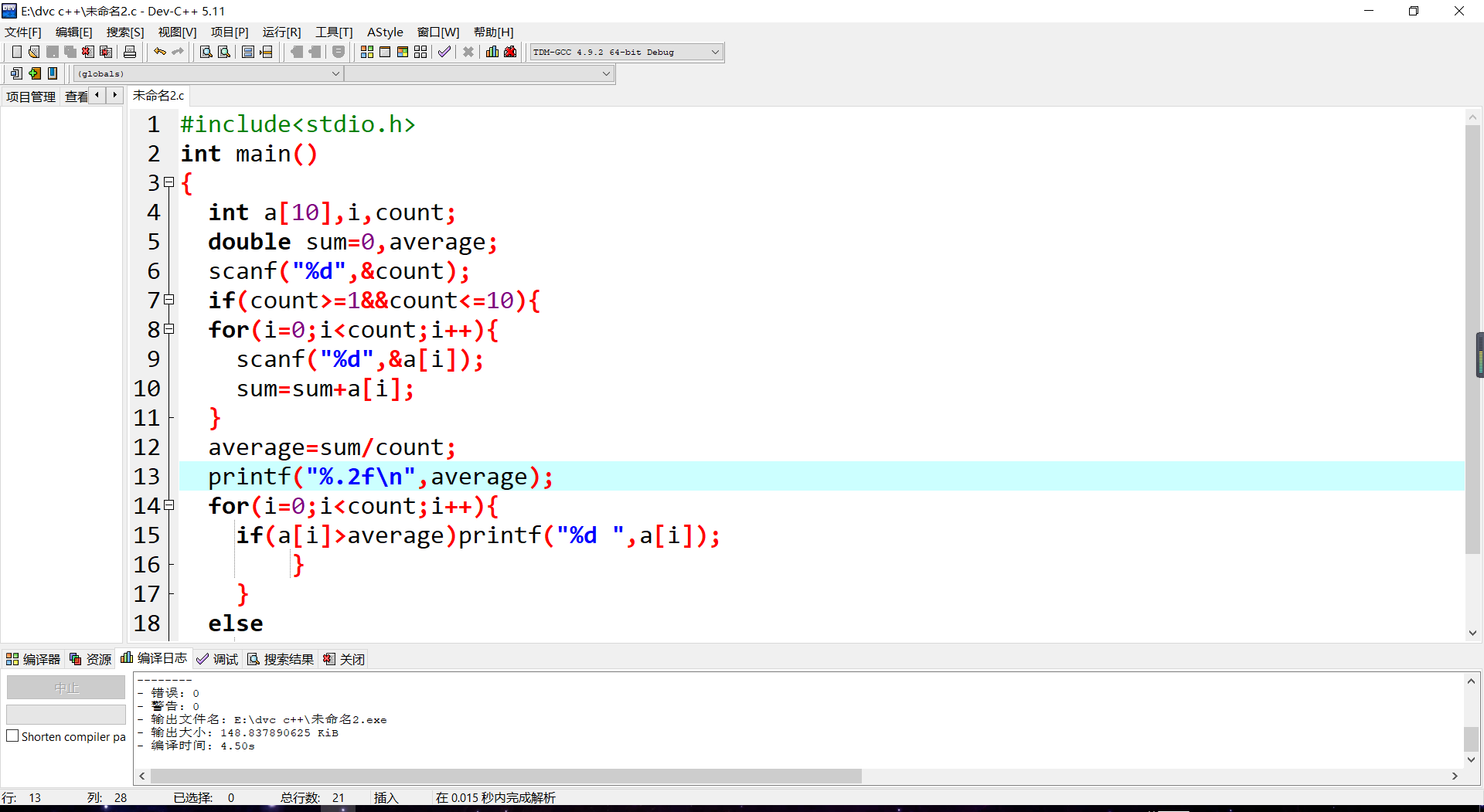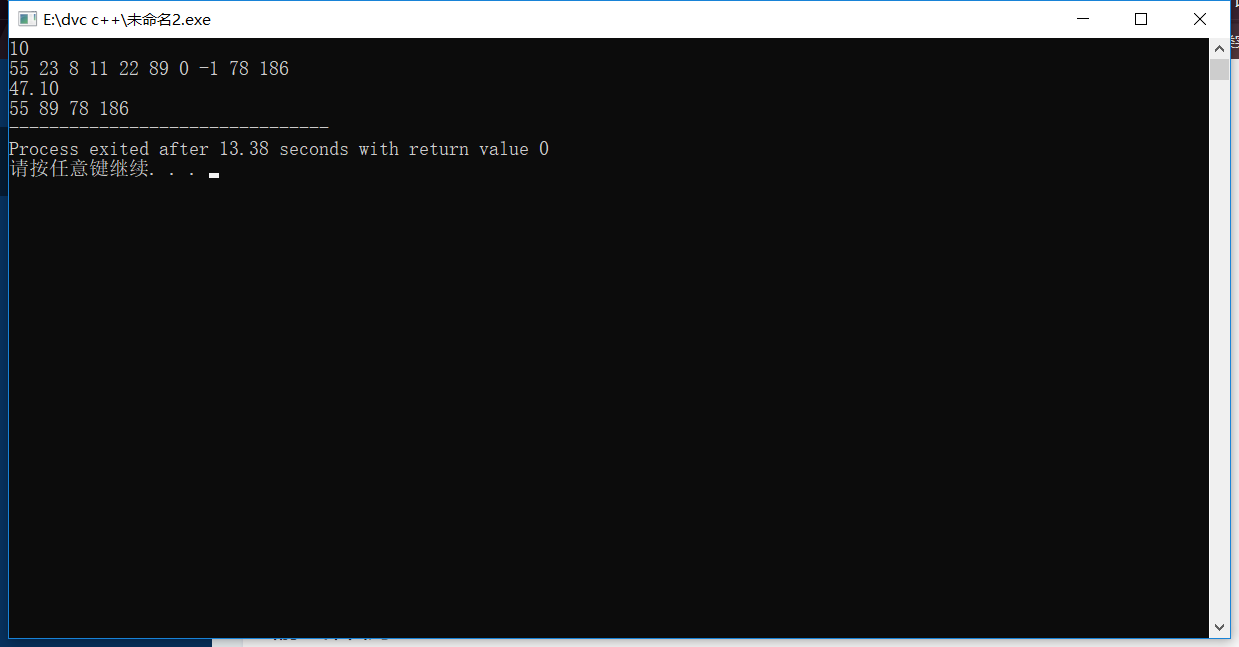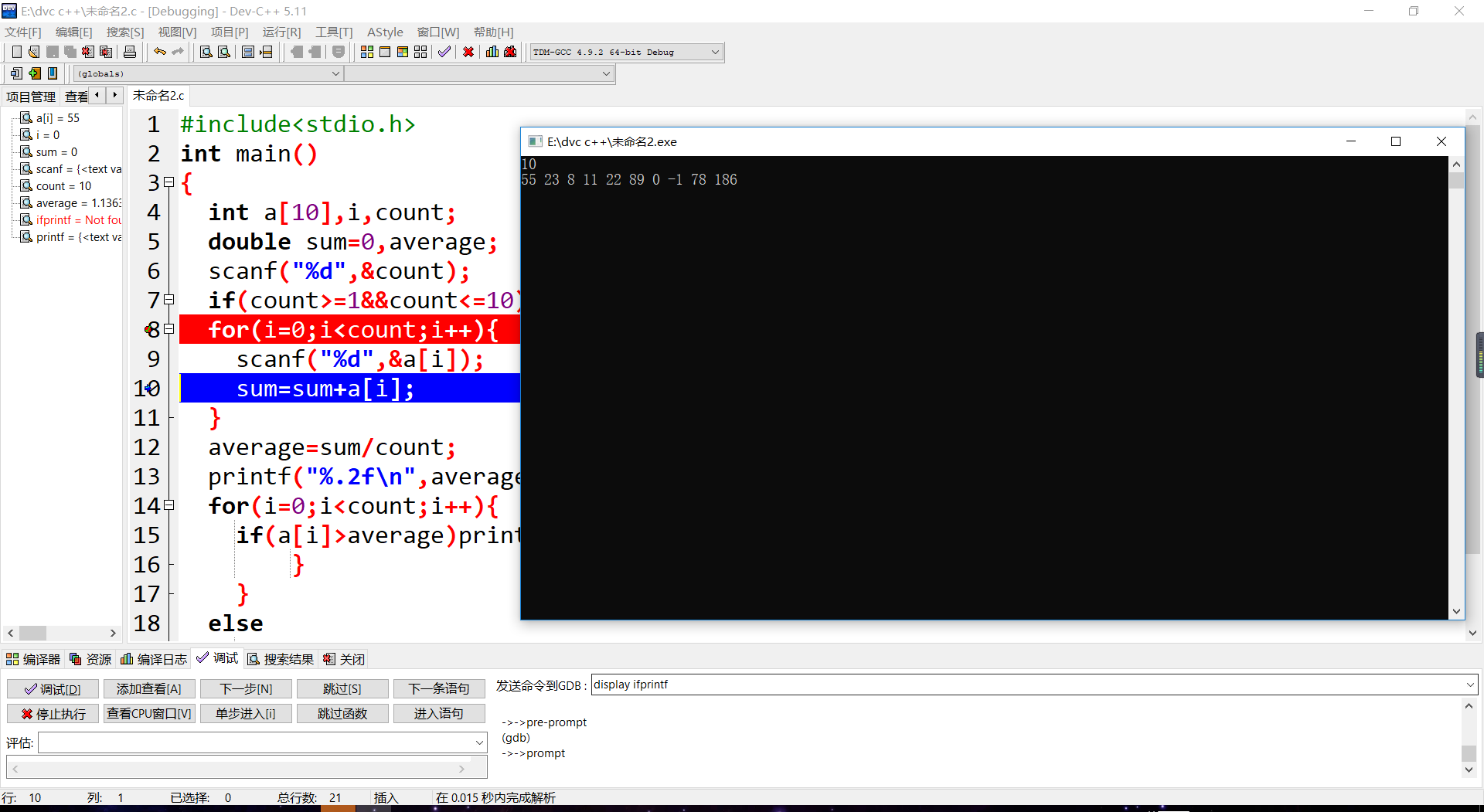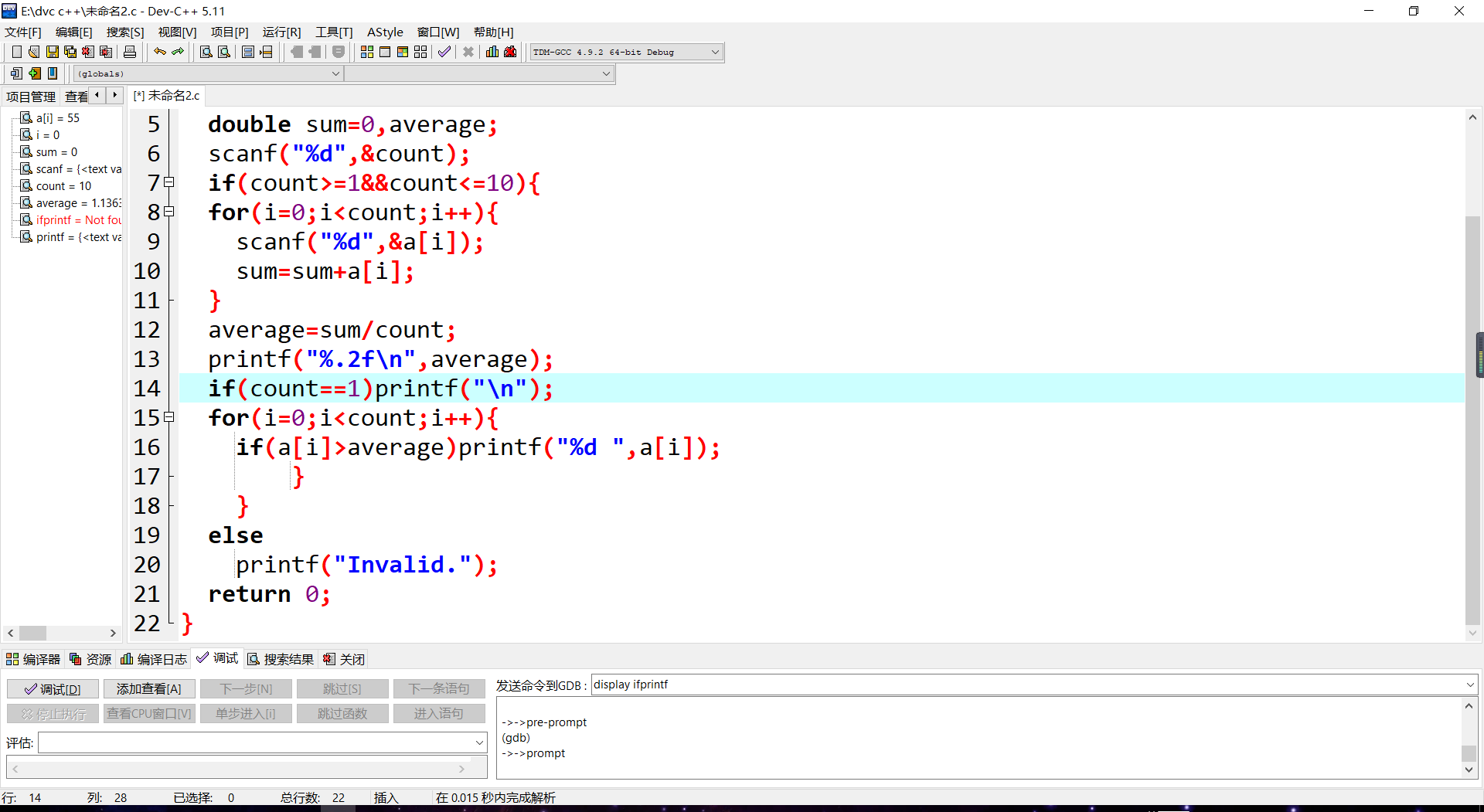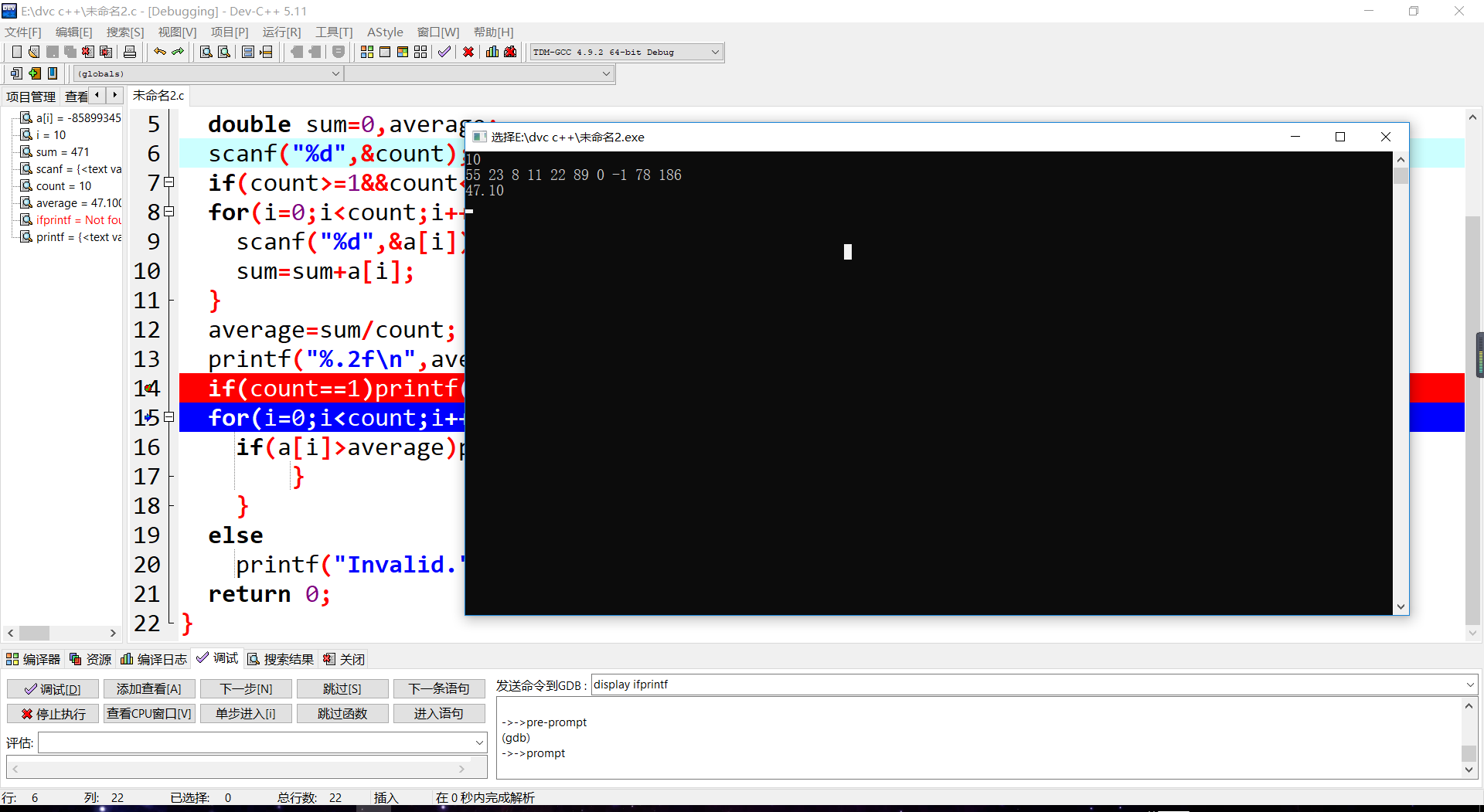第十四次作业 题目 7-2 求最大值及其下标

#include<stdio.h>
int main()
{
int i,a,number,t=0,k=0;
scanf("%d",&number);
for(i=0;i<number;i++){
scanf("%d",&a[i]);
}
t=a;
for(i=0;i<number;i++){
if(a[i]>t){
t=a[i];
k=i;
}
}
printf("%d %d",t,k);
return 0;
}

解题思路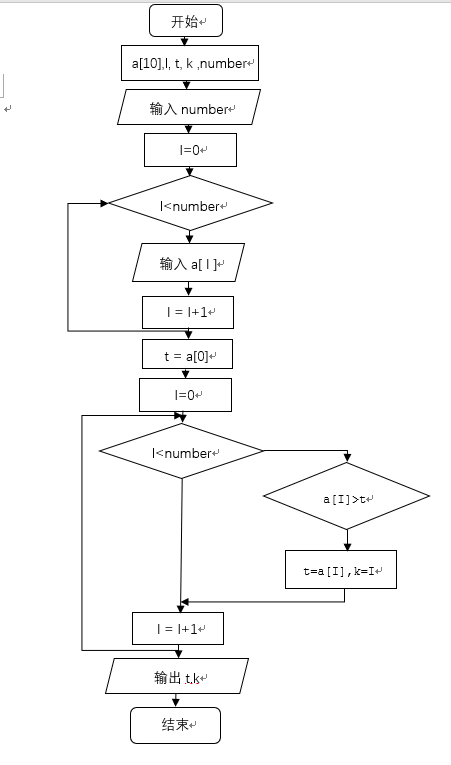题调试过程碰到问题及解决办法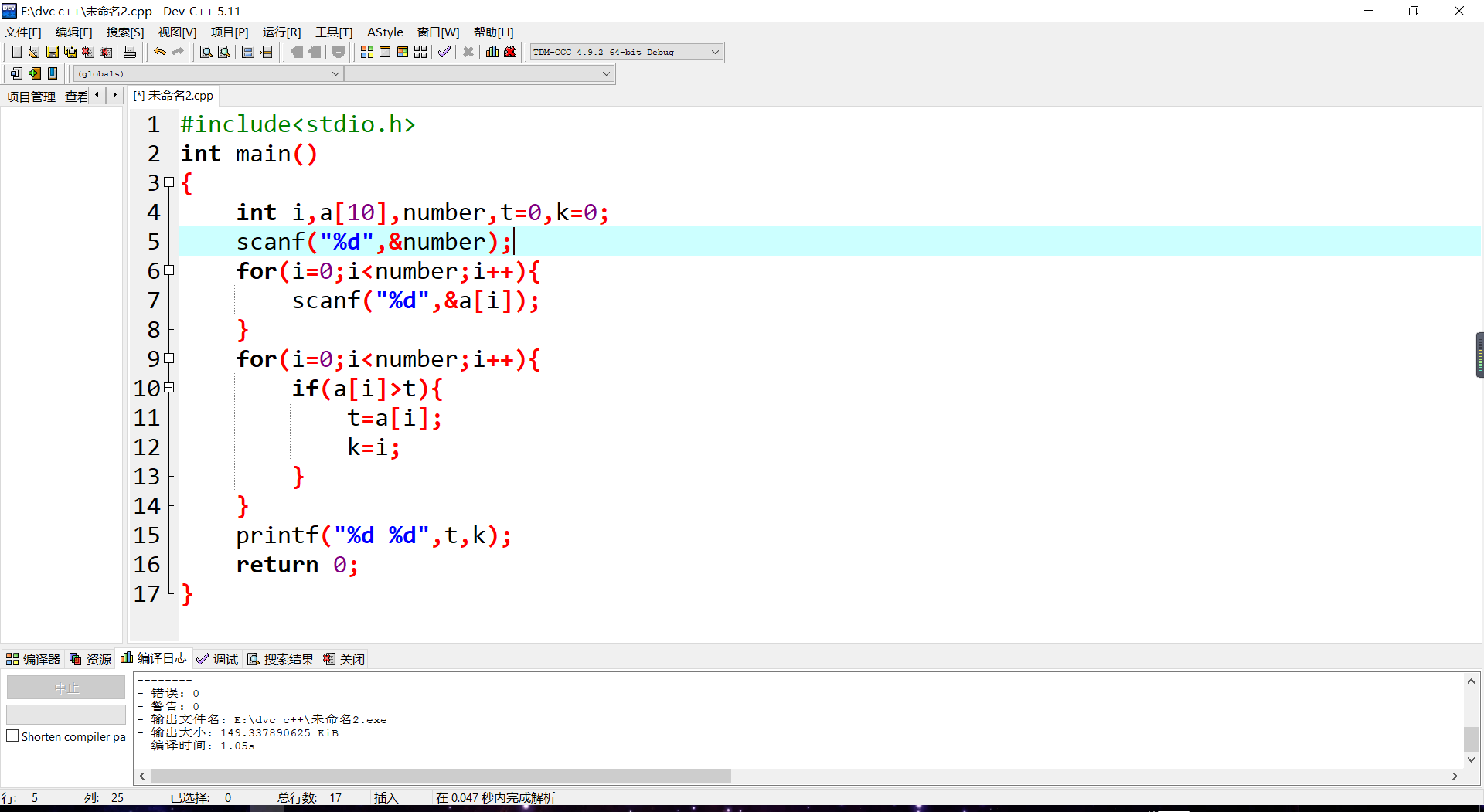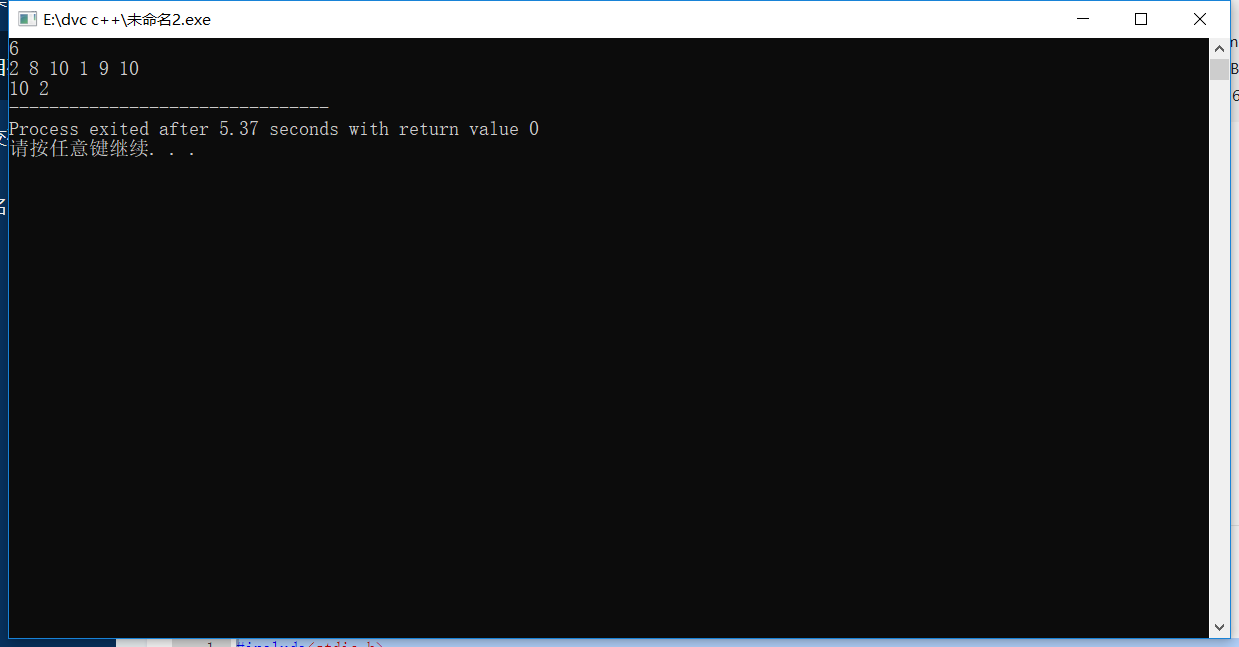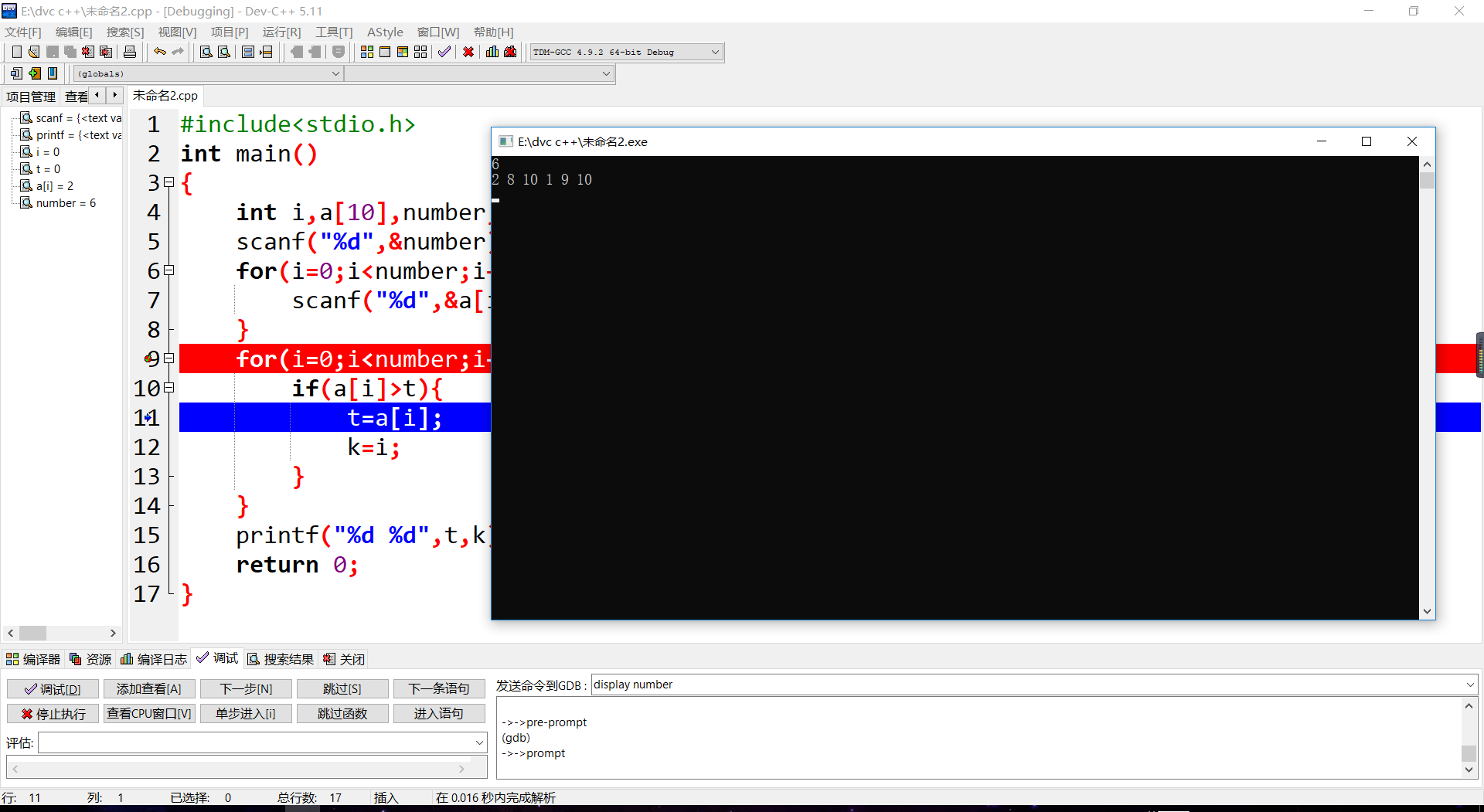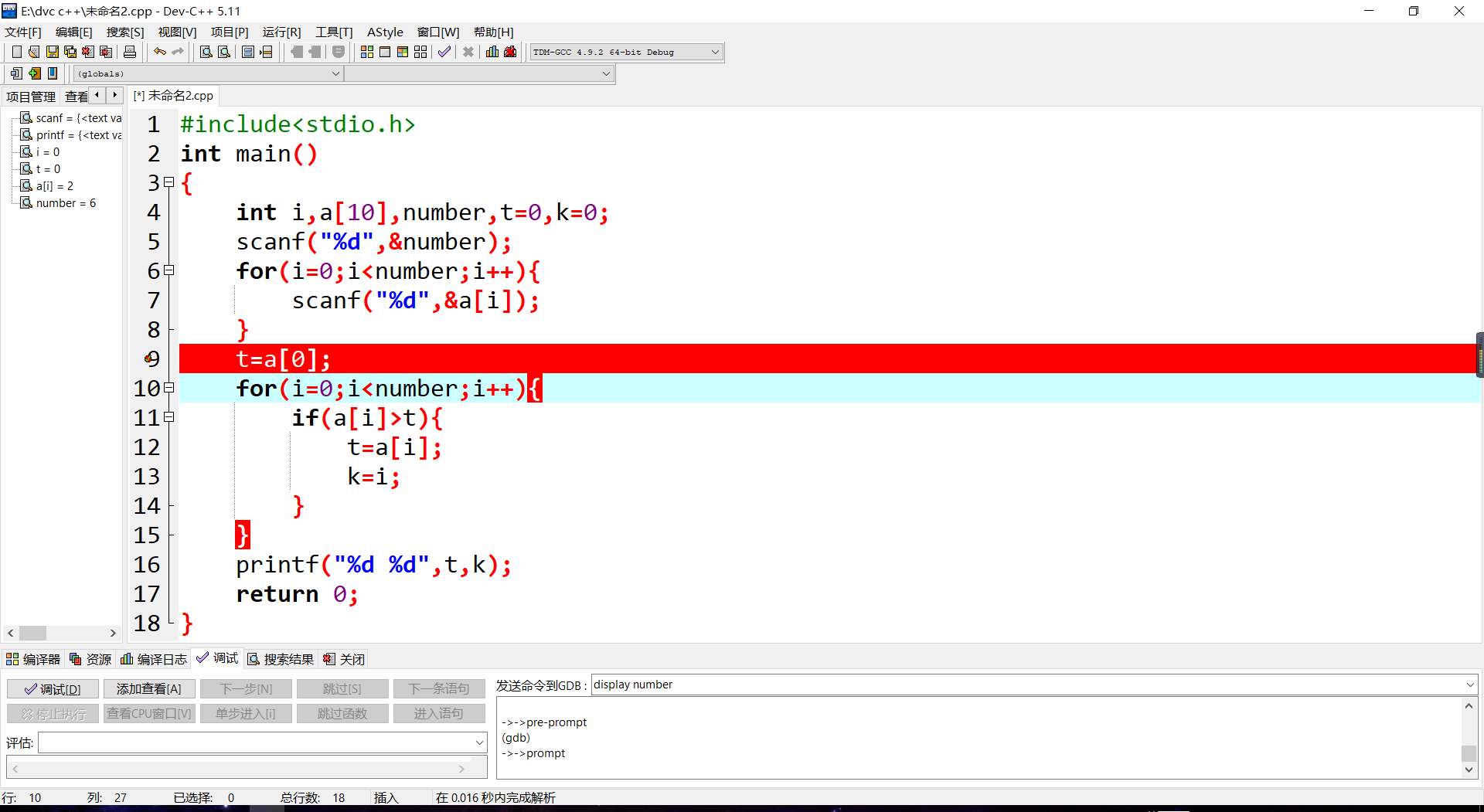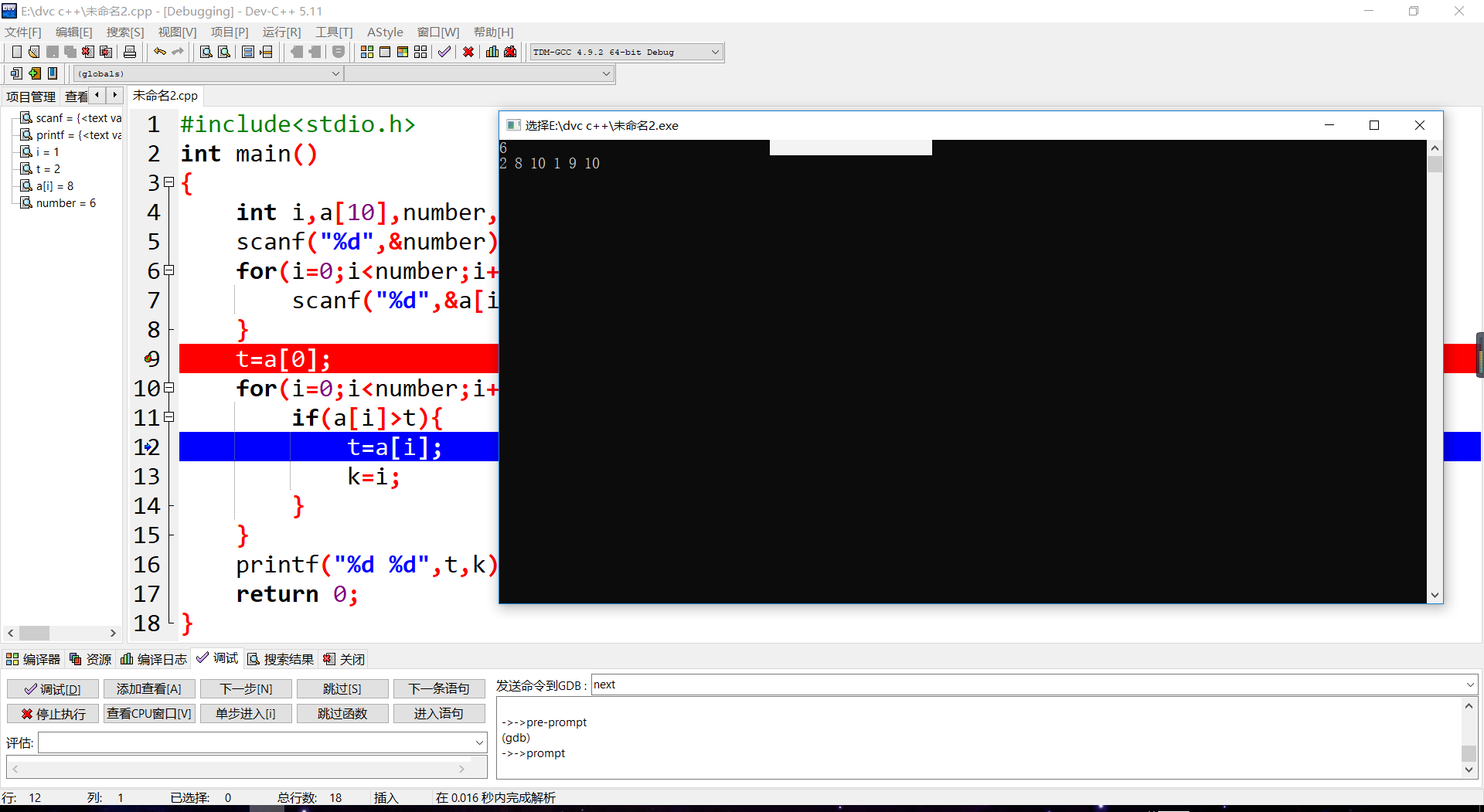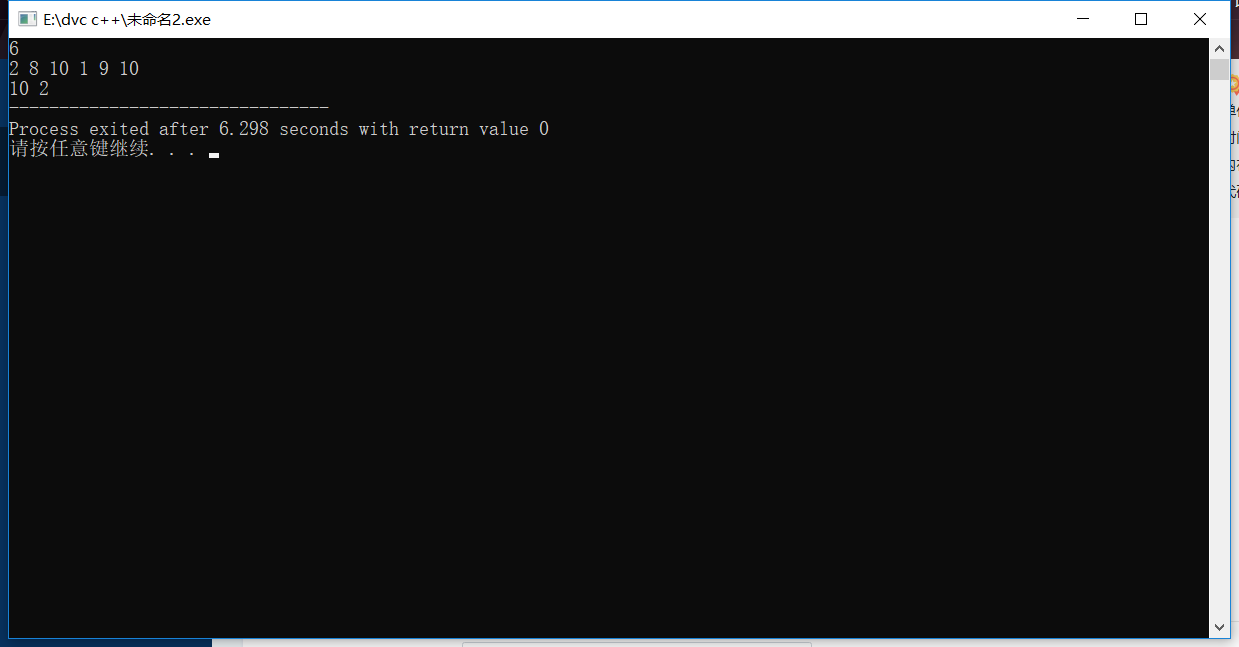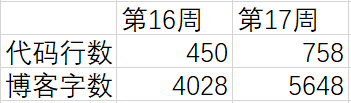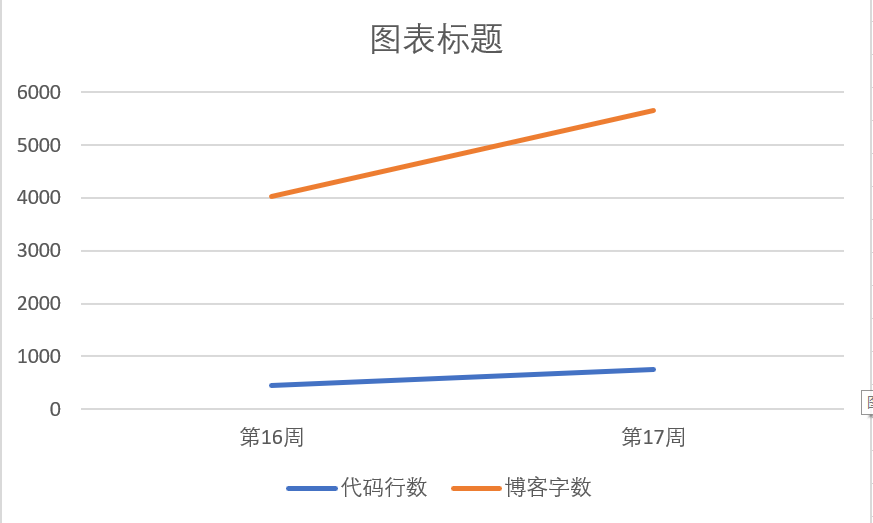posted @ 2018-12-21 17:49  张春宇  阅读(310)  评论(20编辑  收藏  举报